## 为什么调和级数1/n是发散的，而P级数1/n^2是收敛的？

1 观察调和级数$\displaystyle \sum _{n=1}^{\infty }{\frac{1}{n}}$

• $\displaystyle n=10\implies \sum _{n=1}^{10}{\frac{1}{n}}\approx 2.93$
• $\displaystyle n=1000\implies \sum _{n=1}^{1000}{\frac{1}{n}}\approx 7.49$
• $\displaystyle n=100000\implies \sum _{n=1}^{100000}{\frac{1}{n}}\approx 12.09$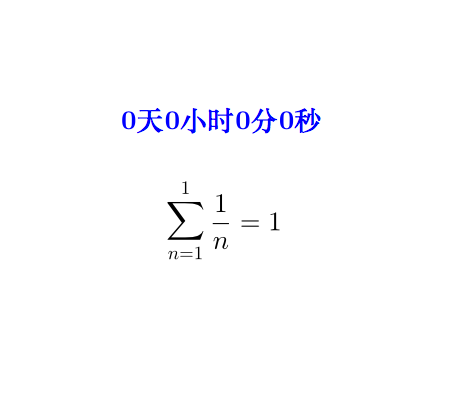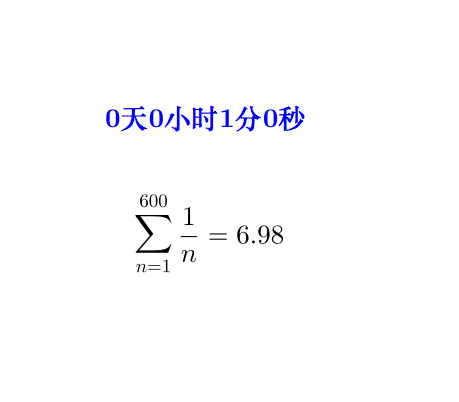4个小时之后：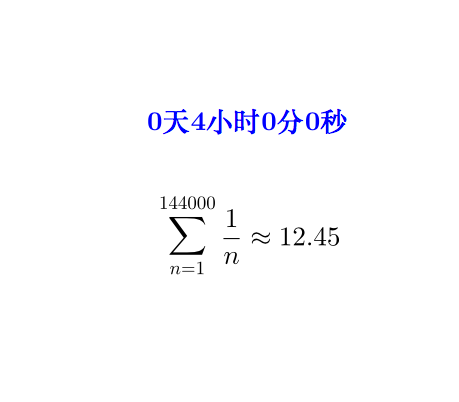6个小时之后：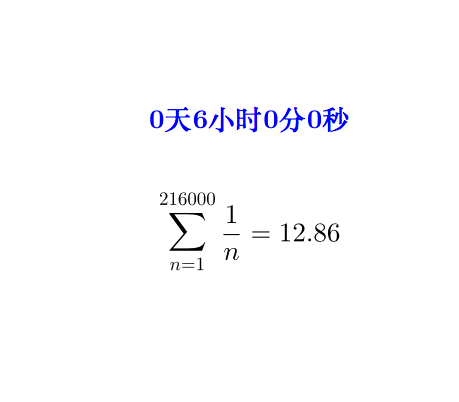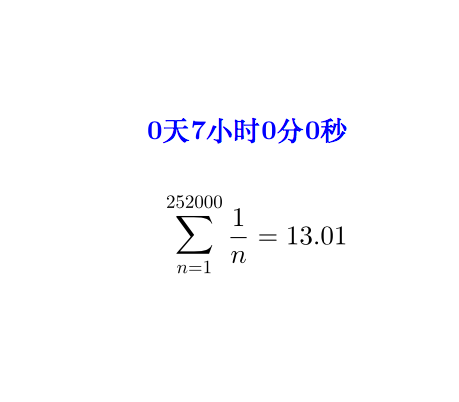$n$越大增长就越慢，按照这个速度，级数和要达到60(没错，就是60这个区区小数)，基本上需要花几十亿年的时间。你盯着屏幕一年、两年，一直盯到你怀疑人生，整数位都一直没有变化，你想或许它收敛了吧，可是它终究在顽强的变大。

Created with GeoGebra

2 收敛还是发散的决定因素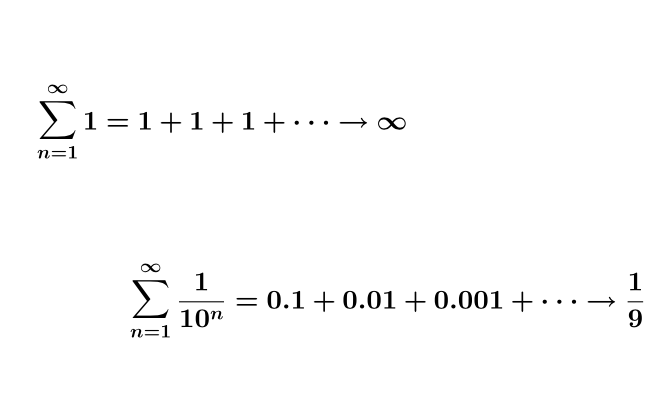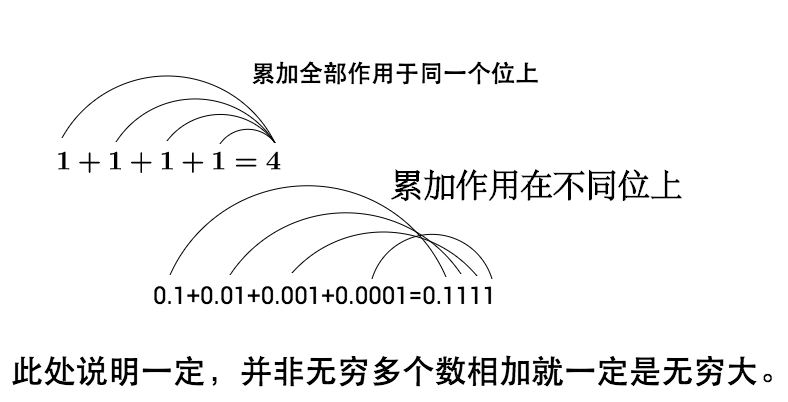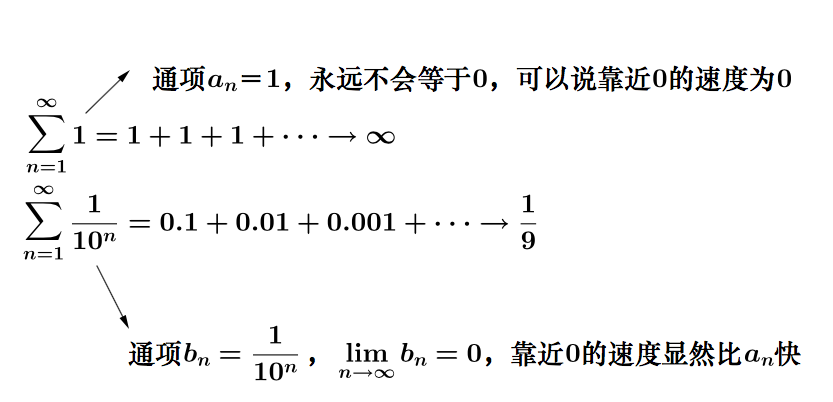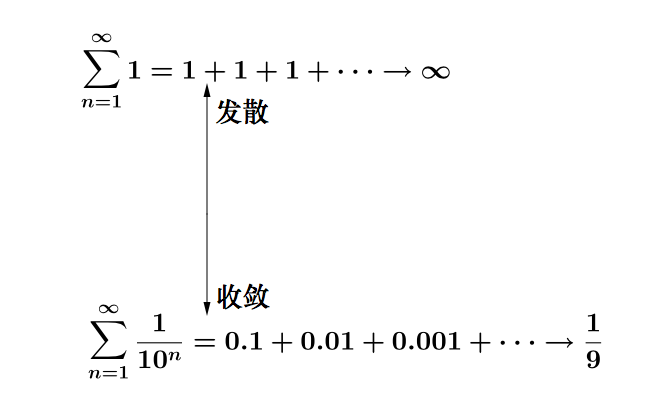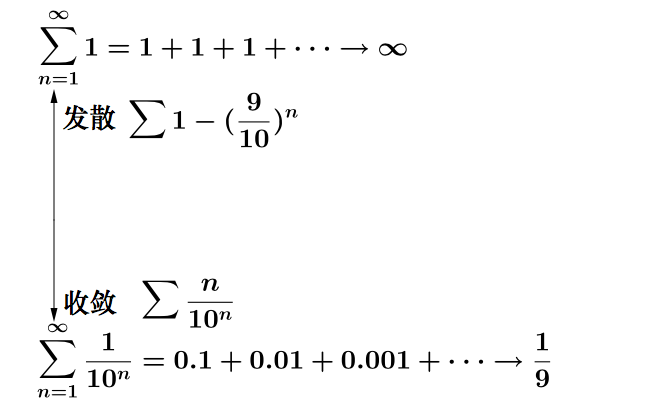3 $\frac{1}{n}$和$\frac{1}{n^2}$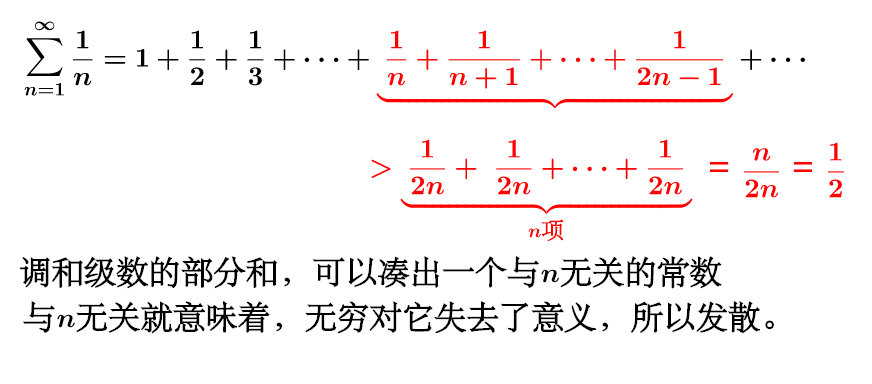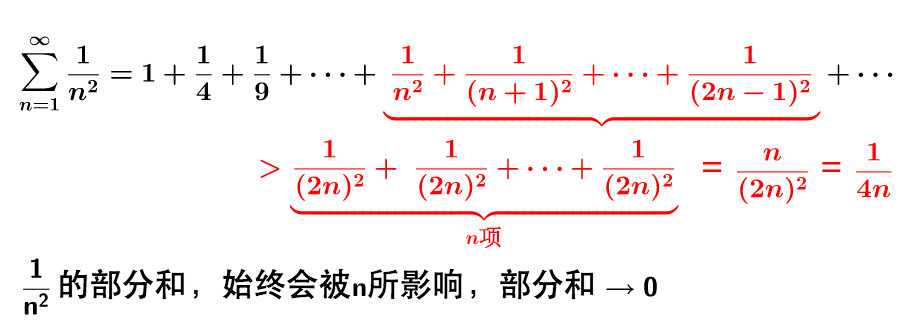4 是否存在一个发散速度最慢的级数？

5 让调和级数收敛

• $n$全部为质数：${1 \over 2}+{1 \over 3}+{1 \over 5}+{1 \over 7}+{1 \over 11}+{1 \over 13}+\cdots \rightarrow \infty$
• $n$为$n!$：${\frac{1}{1}}+{\frac{1}{1}}+{\frac{1}{2}}+{\frac{1}{6}}+{\frac{1}{24}}+{\frac{1}{120}}+\cdots =e$
• $n$为斐波拉契数：${\frac{1}{1}}+{\frac{1}{1}}+{\frac{1}{2}}+{\frac{1}{3}}+{\frac{1}{5}}+{\frac{1}{8}}+\cdots =\psi$

6 结论